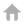Home > Journal Publications

# Research on structural performance evolution of clay based on distribution force model

## Hits:

• Release time:2022-09-04

• Impact Factor:4.5

• DOI number:10.1108/MMMS-09-2021-0158

• Affiliation of Author(s):Emerald

• Journal:Multidiscipline Modeling in Materials and Structures

• Funded by:the National Natural Science Foundation of China [grant numbers: 51778633, 51308552]

• Key Words:Scanning electron microscope; SEM images ; MATLAB image processing; Quantitative statistical parameters; Distribution force model

• Abstract:Purpose The purpose of this paper is to explore the variation law between the clay microstructure and macro external force by using soil scanning electron microscope (SEM) images. Design/methodology/approach First, SEM images of clay were pre-processed by MATLAB, and quantitative statistical parameters such as directional probability entropy, fractal dimension and shape factor are extracted. Second, the distribution force model was proposed, considering that the microscopic parameters of soil particles were independent of each other, and the distribution coefficient was determined according to the analytic hierarchy process (AHP). Then, the fitted formula of quantitative statistical parameters based on the distribution force model was obtained by taking the macroscopic distribution force as independent variable and the microscopic parameters of soil particles as dependent variable. Finally, the correctness of corresponding fitting formula was verified. Findings The results showed that the change of external consolidation pressure has great influence on the directional probability entropy and fractal dimension, while the shape factor reflecting the regular degree of soil particle shape is less sensitive to the consolidation pressure. The fitting formula has high accuracy, and mostly the R value can reach more than 0.9. All the data have passed the test, which proves that the distribution force model proposed in this paper is rational. Originality/value The model can be used to connect the macroscopic stress of soil with the micro-structure deformation of soil particles through mathematical formula, which can provide reference for engineering practice.

• Indexed by:Journal paper

• Discipline:Engineering

• First-Level Discipline:Civil Engineering

• Document Type:J

• ISSN No.:1573-6105

• Translation or Not:no

• Date of Publication:2022-04-04

• Included Journals:SCI

 Central South University  All rights reserved  湘ICP备05005659号-1 Click:MOBILE Version The Last Update Time:..